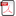### On the Trigonometric Loophole

•  Jirí Stávek

#### Abstract

Two free-will partners A (Alice) and B (Bob) share each a photon from a photon pair emitted from two mutually perpendicularly adjacent non-linear crystals (the spontaneous parametric downconversion SPDC Type I). The third partner C (Claudius) discovered two local hidden trigonometric functions: Cosag (2?) = Cosagitta (2?) = cos2 (?) = ? and Sag (2?) = Sagitta (2?) = sin2 (?) = ? that govern the geometrical (local) probability of correlation and anti-correlation outcomes, respectively. Cosagitta (2?) = cos2 (?) and Sagitta (2?) = sin2 (?) are the heights of an arc in the circle with diameter D = 1. The arc length distance L = ( ? + ?)*D = ( ? ? + ?)*D (D = 1) describes the arc length between the polarization planes of those correlated photons. The angles ?, ? and ?/2 are the angle adjustments of three half-wave plates (HWP) to achieve the tailor-made polarization of those correlated photons. The partner C employs the Claudius’ Theorem to evaluate the joint outcomes found experimentally by the partners A and B. This “trigonometric loophole” can be used to describe the observed and predicted outcomes of correlated particles.

• Full Text:PDF
• DOI:10.5539/apr.v5n6p48This work is licensed under a Creative Commons Attribution 4.0 License.
• ISSN(Print): 1916-9639
• ISSN(Online): 1916-9647
• Started: 2009
• Frequency: bimonthly